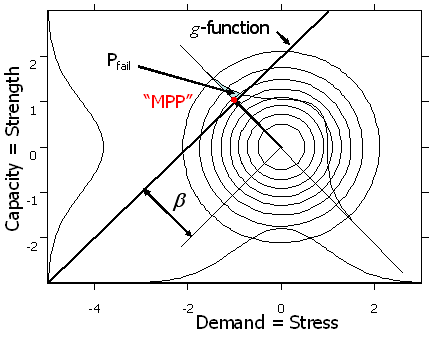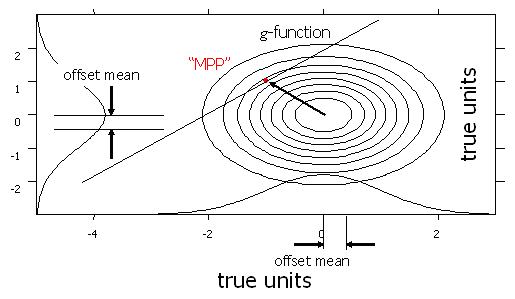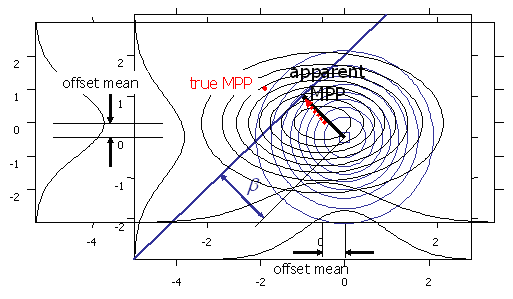## Contrasting the Statistical with the Mathematical Properties of NESSUS/FORM

### The FORM/SORM Paridigm

#### Showing TRUE Mean and Standard Deviation###### Figure 1 The Form/SORM Paradigm

NESSUS/FORM scales the problem according to the sample means and standard deviations, and thus internally the problem always looks as it does in Figure 1. But the true situation is shown in Figure 3, with the dimensions scaled by the true units.

Notice that the true value of the converged MPP(*) (dotted red arrow) is much closer to the true origin (has a higher probability of failure) than the converged MPP (solid blue arrow) because of the location and scaling based on parameter estimates, rather than true, but unknown parameter values.Figure 4 superimposes the situation as seen internally by NESSUS with the true condition.

_____________________

(*) The true value of the converged MPP does not have the anticipated probability of failure that the true MPP (red dot) has. Confusing, isn’t it?